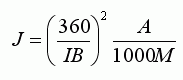I was chatting to ix the other day about mouse movement and this post and I realised something that had been staring me in the face but I'd never given it any thought.

We all know the reasons why when specifying sens it should be in real terms of cm/360, and not just the q3 sensitivity value, which is meaningless on its own. However the same applies to accel. As you can see in the equation, the accel term (circled) is affected by not only cl_mouseaccel, but by many other variables, including mouse dpi and windows sens.This is best illustrated by example.

Take 2 players, X and Y. Player X has 'low' accel (0.1) and player Y has 'high' accel (1.0). Also lets say that player X has a 1600dpi mouse and player Y has a 400dpi mouse. So ok, you probably think that player Y has 10 times more accel than player X, right? Actually, it turns out that player X has the higher acceleration: 60% more accel than player Y.

So, if those with fancy hi-res mice appear to be using 'low' accel, it is not necessarily low in reality.

This presents a problem though, the equivalent sens problem is solved quite easily by specifying in cm/360 terms, but there is no easy way to specify the real accel. So, why not invent one.

If we call the real measure of accel 'J' (i'm running out of letters), and our real measure of sens 'I' (measured with accel off of course). Note that by turning the accel off in the equation above, we get

I := 360/(kSMRB).

Define J to be the full accel term from the equation above (everything multiplying the mouse speed, E), this gives

J := M (kSR)^2 A / 1000.

However, we may not know what k is (if our driver is doing some unknown scaling), or we may not know R accurately. In this case, we can measure 'I' the old-fashioned way and then use the value of I to calculate J:J = Real accel
I = Real sens (cm/360)
B = sensitivity
A = cl_mouseaccel
M = m_yaw

The interpretation of real accel can be thought of as the extra sensitivity given per speed of mouse movement. Units of J depend on whether you use cm or inches for 'I', but for consistency make sure cm are used. J is the number of extra degrees per cm gained by moving the mouse at 1 cm/s. It has units of (deg/cm)/(cm/s) = deg.s/cm^2.

I get a J value of 0.48, so I'm wondering what values others get.